Chapter 8 Part II Hashing Dynamic Hashing Also

• Slides: 16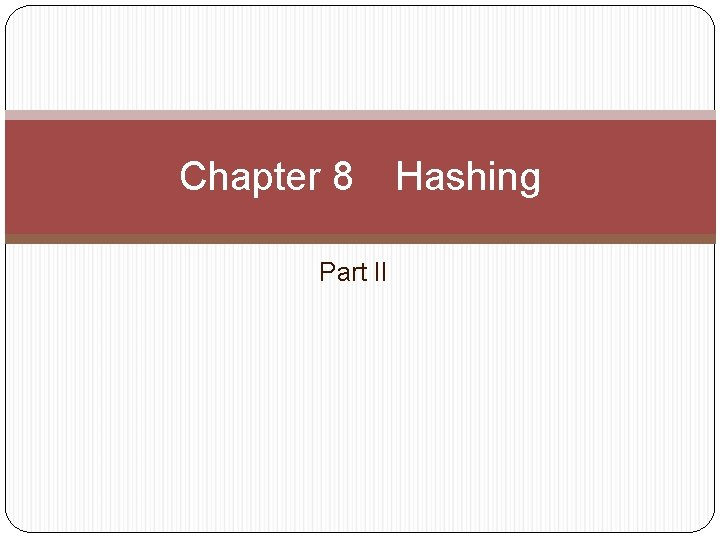Chapter 8 Part II Hashing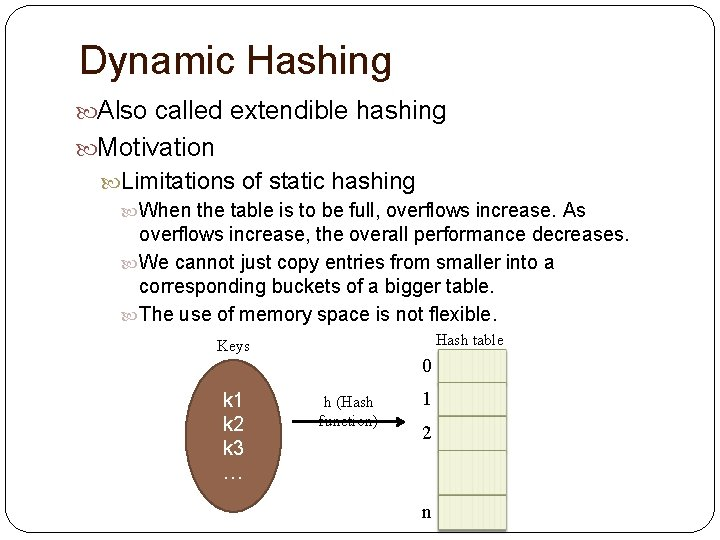Dynamic Hashing Also called extendible hashing Motivation Limitations of static hashing When the table is to be full, overflows increase. As overflows increase, the overall performance decreases. We cannot just copy entries from smaller into a corresponding buckets of a bigger table. The use of memory space is not flexible. Hash table Keys k 1 k 2 k 3 … 0 h (Hash function) 1 2 n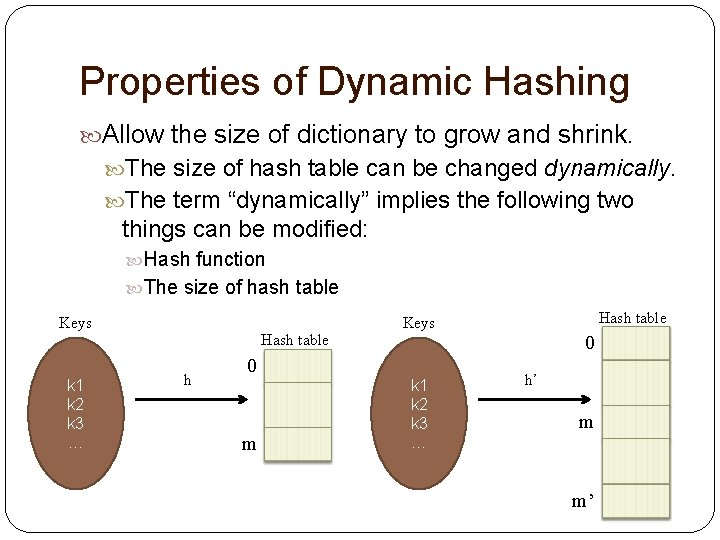Properties of Dynamic Hashing Allow the size of dictionary to grow and shrink. The size of hash table can be changed dynamically. The term “dynamically” implies the following two things can be modified: Hash function The size of hash table Keys k 1 k 2 k 3 … Hash table h 0 m Hash table Keys k 1 k 2 k 3 … 0 h’ m m’8. 3. 2 Dynamic Hashing Using Directories Use an auxilinary table to record the pointer of each bucket. Disk (Directory) Keys Auxilinary table Bucket 1 Bucket 2 k 1 k 2 k 3 … Bucket 3 d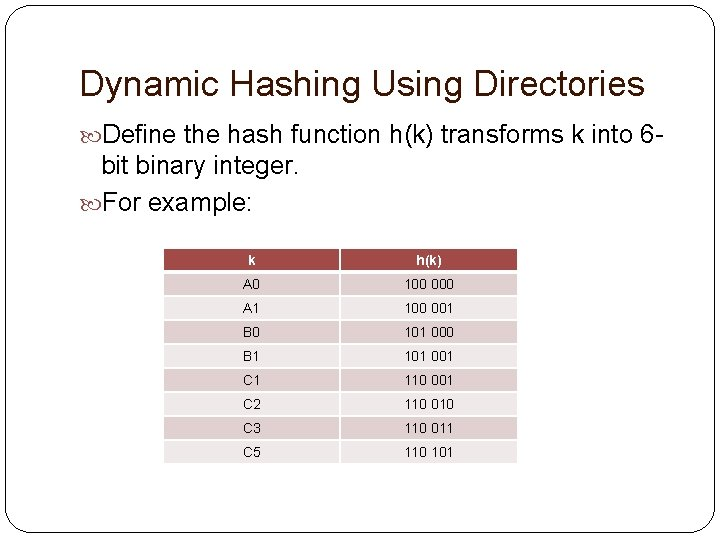Dynamic Hashing Using Directories Define the hash function h(k) transforms k into 6 - bit binary integer. For example: k h(k) A 0 100 000 A 1 100 001 B 0 101 000 B 1 101 001 C 1 110 001 C 2 110 010 C 3 110 011 C 5 110 101Dynamic Hashing Using Directories The size of d is 2 r, where r is the number of bits used to identify all h(x). Initially, Let r = 2. Thus, the size of d = 22 = 4. Suppose h(k, p) is defined as the p least significant bits in h(k), where p is also called dictionary depth. E. g. h(C 5) = 110 101 h(C 5, 2) = 01 h(C 5, 3) = 101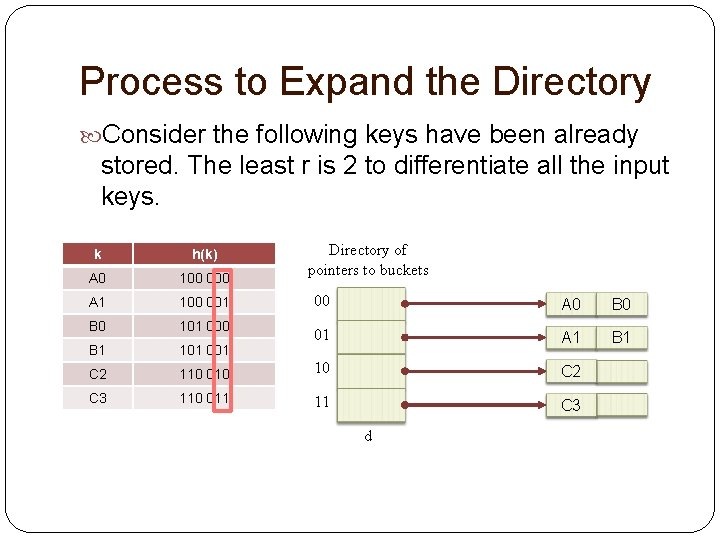Process to Expand the Directory Consider the following keys have been already stored. The least r is 2 to differentiate all the input keys. k h(k) A 0 100 000 A 1 100 001 B 0 101 000 B 1 101 001 C 2 C 3 Directory of pointers to buckets 00 A 0 B 0 01 A 1 B 1 110 010 10 C 2 110 011 11 C 3 d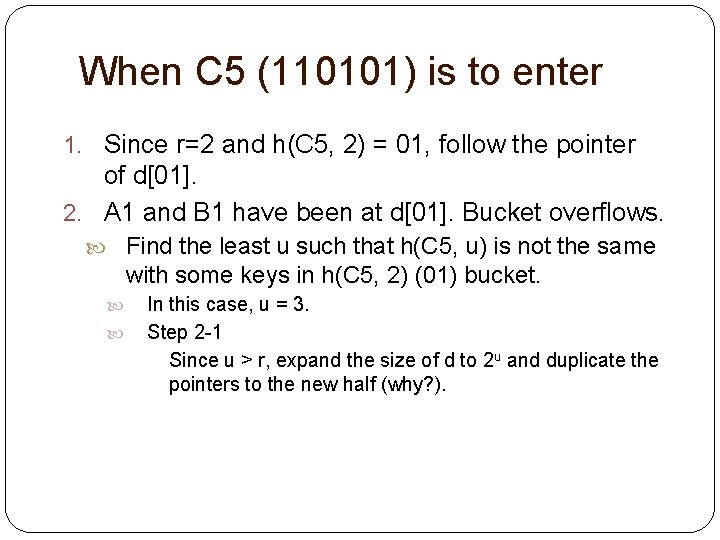When C 5 (110101) is to enter 1. Since r=2 and h(C 5, 2) = 01, follow the pointer of d. 2. A 1 and B 1 have been at d. Bucket overflows. Find the least u such that h(C 5, u) is not the same with some keys in h(C 5, 2) (01) bucket. In this case, u = 3. Step 2 -1 Since u > r, expand the size of d to 2 u and duplicate the pointers to the new half (why? ).When C 5 (110101) is to enter Table的size變大後照理說每個entry都必須重新根據新的hash function重算其所在的bucket。但由於重算耗費的時間太多，所 以暫時保留在原來的bucket，在新的bucket中保留舊的pointer， 直到下次出現overflow時再重算。 000 A 0 B 0 001 A 1 B 1 010 C 2 011 C 3 100 101 110 111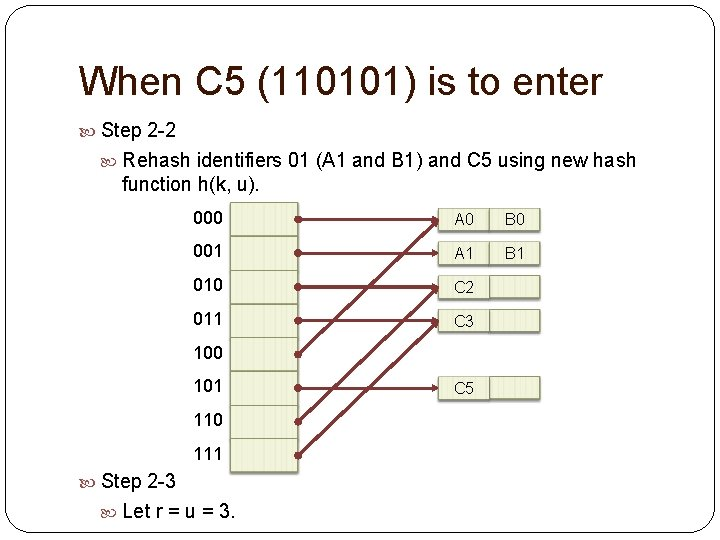When C 5 (110101) is to enter Step 2 -2 Rehash identifiers 01 (A 1 and B 1) and C 5 using new hash function h(k, u). 000 A 0 B 0 001 A 1 B 1 010 C 2 011 C 3 100 101 110 111 Step 2 -3 Let r = u = 3. C 5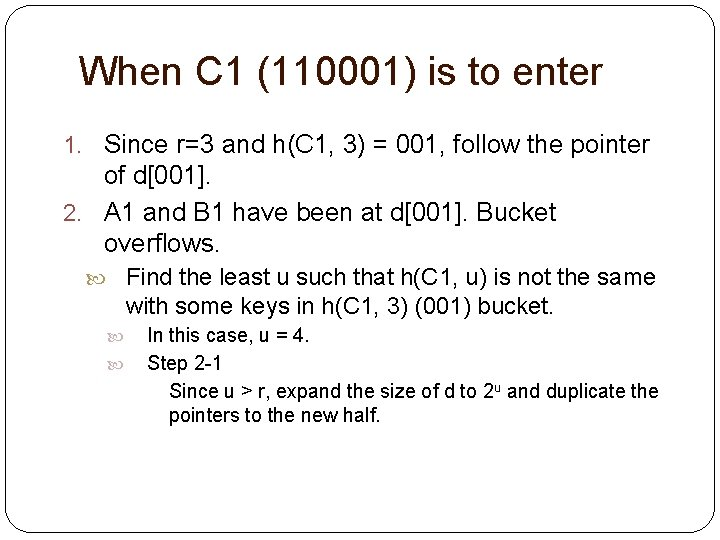When C 1 (110001) is to enter 1. Since r=3 and h(C 1, 3) = 001, follow the pointer of d. 2. A 1 and B 1 have been at d. Bucket overflows. Find the least u such that h(C 1, u) is not the same with some keys in h(C 1, 3) (001) bucket. In this case, u = 4. Step 2 -1 Since u > r, expand the size of d to 2 u and duplicate the pointers to the new half.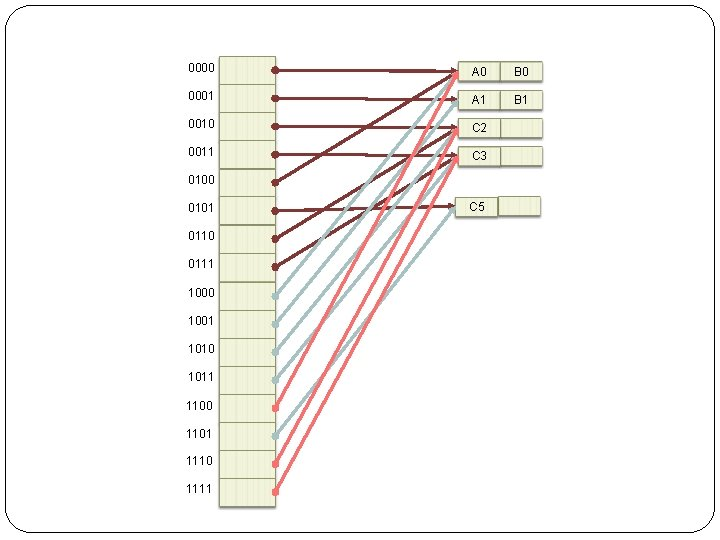0000 A 0 B 0 0001 A 1 B 1 0010 C 2 0011 C 3 0100 0101 0110 0111 1000 1001 1010 1011 1100 1101 1110 1111 C 5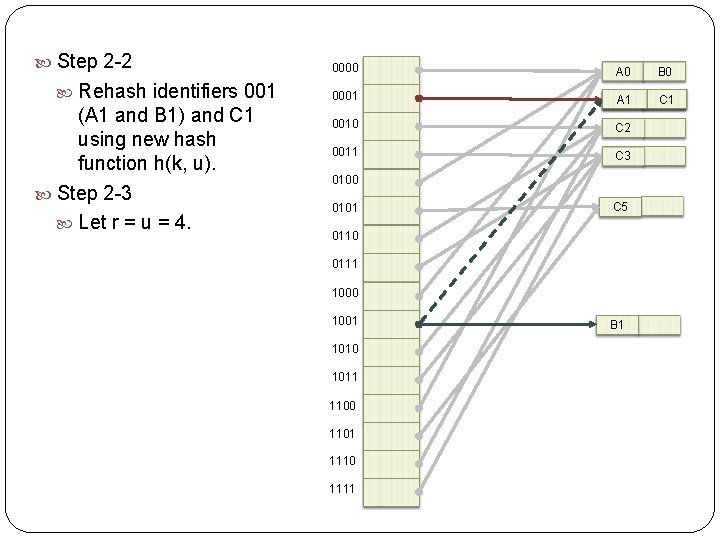Step 2 -2 Rehash identifiers 001 (A 1 and B 1) and C 1 using new hash function h(k, u). Step 2 -3 Let r = u = 4. 0000 A 0 B 0 0001 A 1 C 1 0010 C 2 0011 C 3 0100 0101 C 5 0110 0111 1000 1001 1010 1011 1100 1101 1110 1111 B 1When C 4 (110100) is to enter 1. Since r=4 and h(C 4, 4) = 0100, follow the pointer of d. 2. A 0 (100000) and B 0 ((101000)) have been at d. Bucket overflows. Find the least u such that h(C 1, u) is not the same with some keys in h(C 1, 4) (0100) bucket. In this case, u = 3. Step 2 -1 Since u = 3 < r = 4, d is not required to expand its size.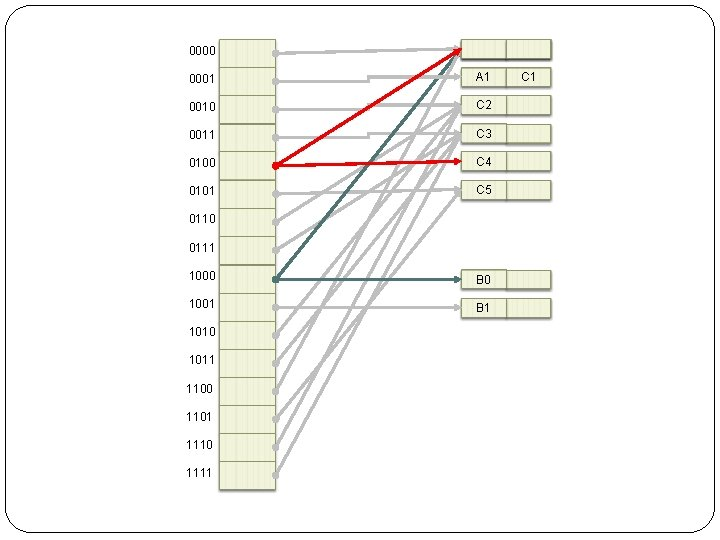0000 A 0 B 0 0001 A 1 C 1 0010 C 2 0011 C 3 0100 C 4 0101 C 5 0110 0111 1000 B 0 1001 B 1 1010 1011 1100 1101 1110 1111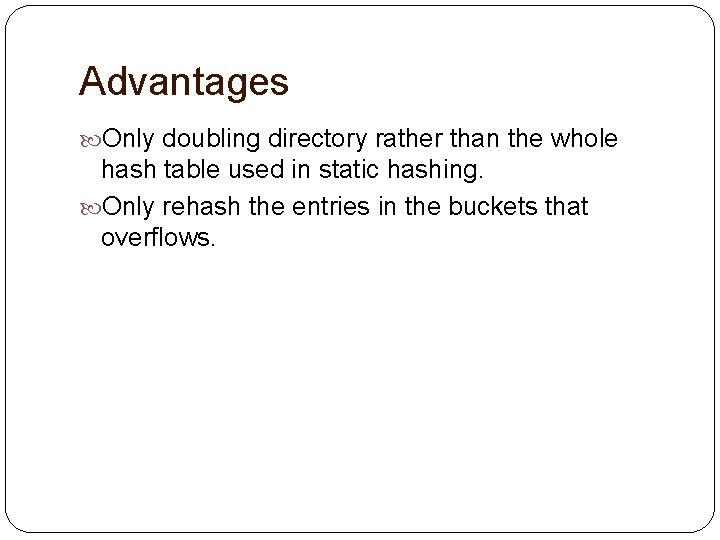Advantages Only doubling directory rather than the whole hash table used in static hashing. Only rehash the entries in the buckets that overflows.